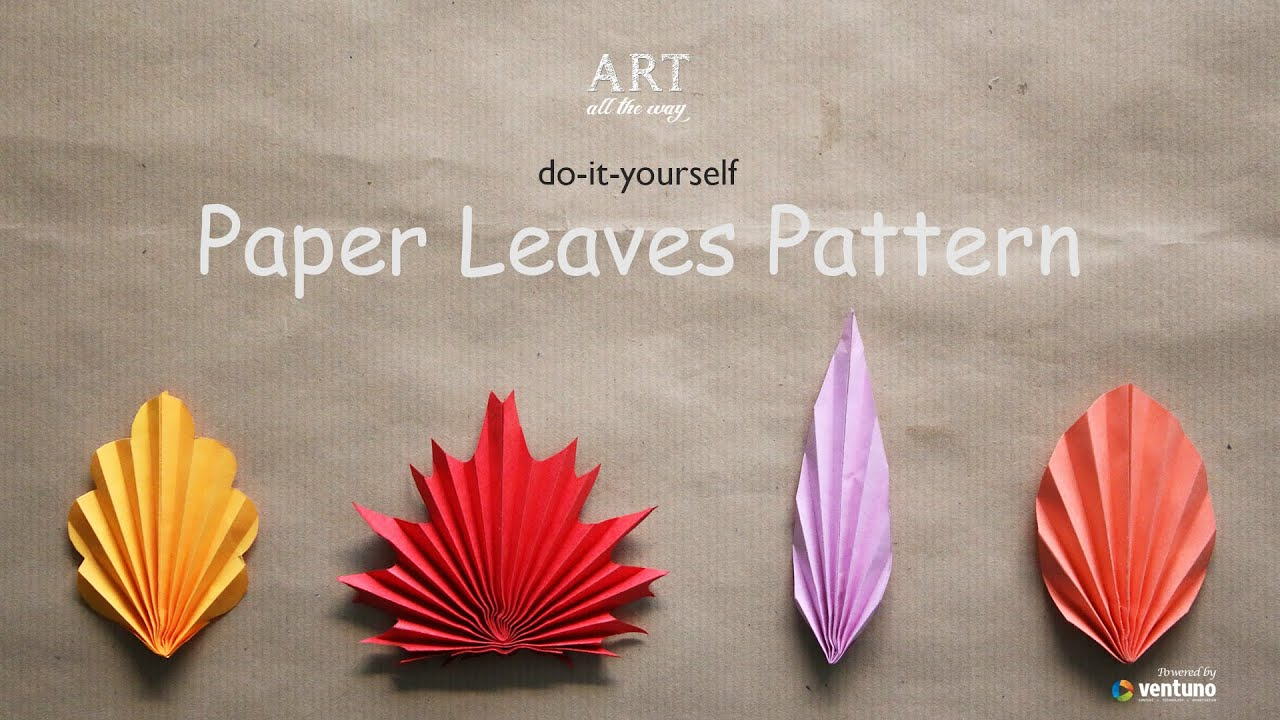# DIY : Paper Leaves Pattern

ArtYou can easily make Paper Leaves Pattern like that. Check out this video for step by step instruction.

DIY : Paper Leaves Pattern Steps : Leaf Pattern : 1
1) Take a rectangular sheet of 15X7.5 cms.
2) Fold in to half horizontally then draw a curve.
3) Use the scissor and cut along the curve.
4) Open the sheet and make pre crease using a scale and used ball point tip.
5) Start making crimps as shown.
6) Now fold the crimped sheet as shown.
7) Stick with glue and thats it!

Leaf Pattern : 2
1) Take a lengthy sheet of 20X5 cms.
2) Fold in to half horizontally.
3) Using a scale and pencil draw a diagonal line and cut along the line.
4) Open the sheet and make pre crease using a scale and used ball point tip.
5) Start making crimps as shown.
6) Now fold the crimped sheet as shown.
7) Stick with glue and thats it!

Leaf Pattern : 3
1) Take a rectangular sheet of 15X7.5 cms.
2) fold in to half and draw like shown above.
3) Open the sheet and make pre crease using a scale and used ball point tip.
4) Start making crimps as shown.
5) Now fold the crimped sheet as shown.
6) Stick with glue and thats it!

Leaf Pattern : 4
1) Take a square sheet of 15X15 cms.
2) Fold in to half like shown.
3) Draw random curves with sharp ends away fron the centre.
4) Open the sheet and make pre crease using a scale and used ball point tip.
5) Start making crimps as shown.
6) Now fold the crimped sheet as shown.
7) Stick with glue and thats it!